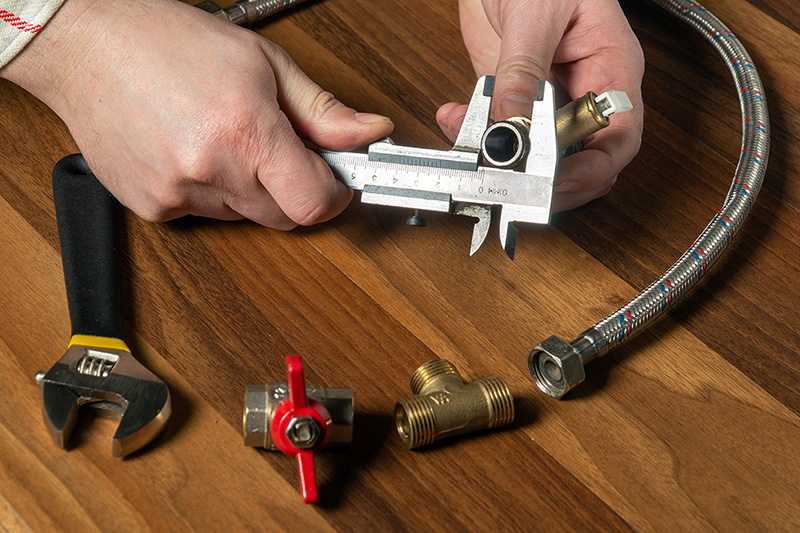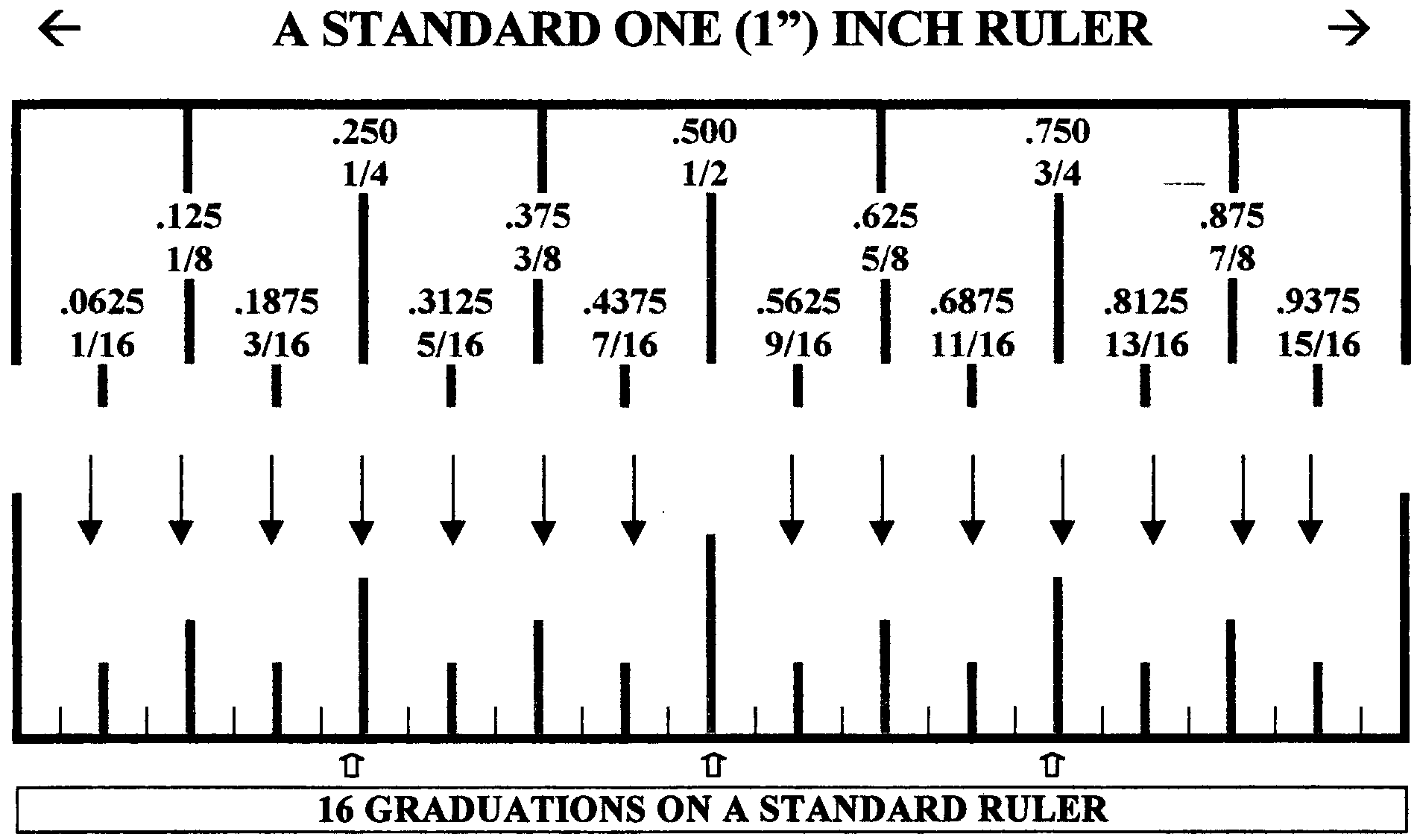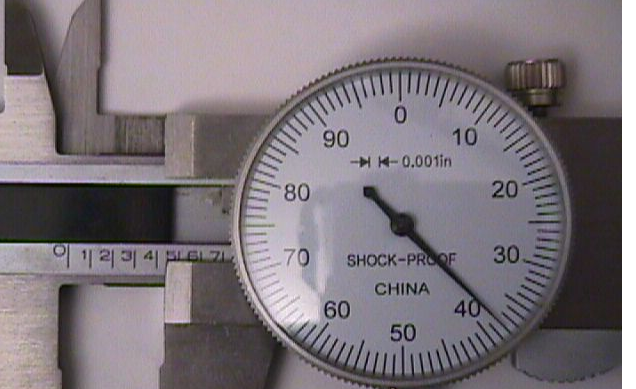# Why a Dial Caliper is more accurate than a Ruler

By Marketing November 19, 2021Calipers can measure accuracy to one-hundredth of a millimeter and one thousand of an inch.

Proper Dial Caliper reading made easy.  Fractions, Decimals, Dash Sizing can be made easy with a Dial Caliper.

It can measure ID (inside diameter) and OD (outside diameter).  These calipers have a dial that displays the measurement with an indicator needle. The dial records the final fraction of a millimeter or inch. This measurement is then combined with the reading given on the scale running along the beam of the caliper.

Let’s begin with a Ruler, it is divided into tens of an inch.

Therefore, each line on the Ruler represents 0.10 inches

On the Ruler, every ten lines is marked with the number of inches

1” = Sum of 10 lines each representing 0.10 inches

1” = 10 x (0.10)Dial is divided into thousands of an inch. Therefore, each line on the Dial represents 0.001 inches.

On the Dial, every ten lines is marked with the hundreds of an inch

0.010” = Sum of 10 lines each representing 0.001 inches

0.010” = 10 x (0.001)

At each hundred of an inch, the Dial is marked with a whole number

10 = 0.010

20 = 0.020

30 = 0.030

etc.NOTE:  Measurements made with calipers should be within 5/1000 or 0.005 of the dimensions specified by the manufacturer.  If your measurement is greater or less than the manufacturer recommendation ±0.005”, please repeat your measurement and review the assembly procedures for possible errors.

Reading the dial caliper is a two-step process:

• Zero out the dial: close the jaws and check that the indicator points to "0" on the dial face.
• Adjust the jaws to measure the object.
• First, look at the sliding jaw and note the last main scale graduation that it passed.
• Next, look at the dial and determine which number the hand is pointing.